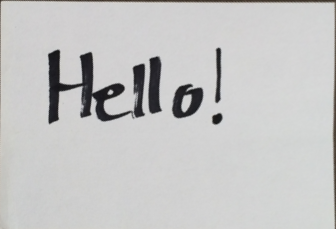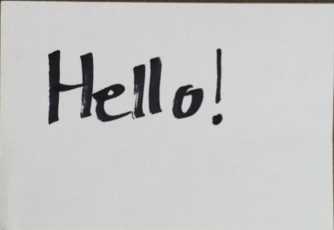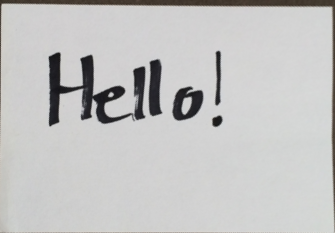# Perspective Examples¶

:

from easycv import Image, Pipeline
from easycv.transforms import Perspective, Select


For this example we will load an example image from the images folder.

:

img = Image('images/hello.png')
img

:## Perspective¶

To execute a perspective transform we just apply the Prespective Transform with the desired points to the image, for more information check the reference.

:

points = [(71, 238), (354, 114), (470, 257), (186, 437)] # corners of the paper

img.apply(Perspective(points=points))

:We could also select the points with the Select Transform, for more information check the reference.

:

points = img.apply(Select.point(n=4))["points"]
points

:

[(71, 238), (352, 114), (470, 258), (187, 437)]


And then call as we did in the last example.

:

img.apply(Perspective(points=points))

:We can also create a pipeline that selects and applies the perspective transform.

:

pipe = Pipeline([Select.point(n=4), Perspective()])
pipe

:

Pipeline (pipeline) with 2 transforms
1: Select (method=point, n=4)
2: Perspective (method=(...), points=(...))


This will open a window asking to select the points and then execute a perspective transform on the selected points.

:

img.apply(pipe)

: